## T-Test: Dr. Semmelweis and the discovery of handwashing

This article only illustrates the use of t-test in a real life problem but does not provide any technical information on what is T-Test or how T-Test works. I will go through the T-test in details in another post and will link it into this post.

## Intro

I was looking for a cool dataset to illustrate the use of T.test and I found this DataCamp project “Dr. Semmelweis and the discovery of handwashing”. This a straightforward project but I really like the way they introduce it and specifically how they show beyond doubt that statistic plays a vital role in the medical field.

Here is the discovery of the Dr.Ignaz Semmelweis:
“In 1847 the Hungarian physician Ignaz Semmelweis makes a breakthough discovery: He discovers handwashing. Contaminated hands was a major cause of childbed fever and by enforcing handwashing at his hospital he saved hundreds of lives.”

## 1. Meet Dr. Ignaz Semmelweis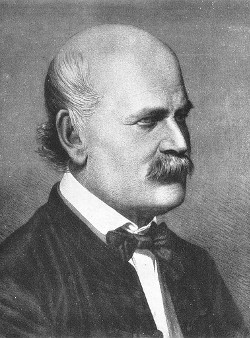This is Dr. Ignaz Semmelweis, a Hungarian physician born in 1818 and active at the Vienna General Hospital. If Dr. Semmelweis looks troubled it’s probably because he’s thinking about childbed fever: A deadly disease affecting women that just have given birth. He is thinking about it because in the early 1840s at the Vienna General Hospital as many as 10% of the women giving birth die from it. He is thinking about it because he knows the cause of childbed fever: It’s the contaminated hands of the doctors delivering the babies. And they won’t listen to him and wash their hands!

In this notebook, we’re going to reanalyze the data that made Semmelweis discover the importance of handwashing. Let’s start by looking at the data that made Semmelweis realize that something was wrong with the procedures at Vienna General Hospital.

# Load in the tidyverse package
library(tidyverse)
library(ggplot2)
# Print out yearly
yearly

year births deaths clinic
1841 3036 237 clinic 1
1842 3287 518 clinic 1
1843 3060 274 clinic 1
1844 3157 260 clinic 1
1845 3492 241 clinic 1
1846 4010 459 clinic 1
1841 2442 86 clinic 2
1842 2659 202 clinic 2
1843 2739 164 clinic 2
1844 2956 68 clinic 2
1845 3241 66 clinic 2
1846 3754 105 clinic 2

## 2. The alarming number of deaths

The table above shows the number of women giving birth at the two clinics at the Vienna General Hospital for the years 1841 to 1846. You’ll notice that giving birth was very dangerous; an alarming number of women died as the result of childbirth, most of them from childbed fever.

We see this more clearly if we look at the proportion of deaths out of the number of women giving birth.

# Adding a new column to yearly with proportion of deaths per no. births
yearly$proportion_deaths&lt;-yearly$deaths/yearly$births # Print out yearly yearly  year births deaths clinic proportion_deaths 1841 3036 237 clinic 1 0.07806324 1842 3287 518 clinic 1 0.15759051 1843 3060 274 clinic 1 0.08954248 1844 3157 260 clinic 1 0.08235667 1845 3492 241 clinic 1 0.06901489 1846 4010 459 clinic 1 0.11446384 1841 2442 86 clinic 2 0.03521704 1842 2659 202 clinic 2 0.07596841 1843 2739 164 clinic 2 0.05987587 1844 2956 68 clinic 2 0.02300406 1845 3241 66 clinic 2 0.02036409 1846 3754 105 clinic 2 0.02797017 ## 3. Death at the clinics If we now plot the proportion of deaths at both clinic 1 and clinic 2 we’ll see a curious pattern… # Setting the size of plots in this notebook options(repr.plot.width=7, repr.plot.height=4) # Plot yearly proportion of deaths at the two clinics ggplot(data=yearly, aes(x=year, y=proportion_deaths, group=clinic, color=clinic)) + geom_line() + geom_point()+ scale_color_brewer(palette="Paired")+ theme_minimal()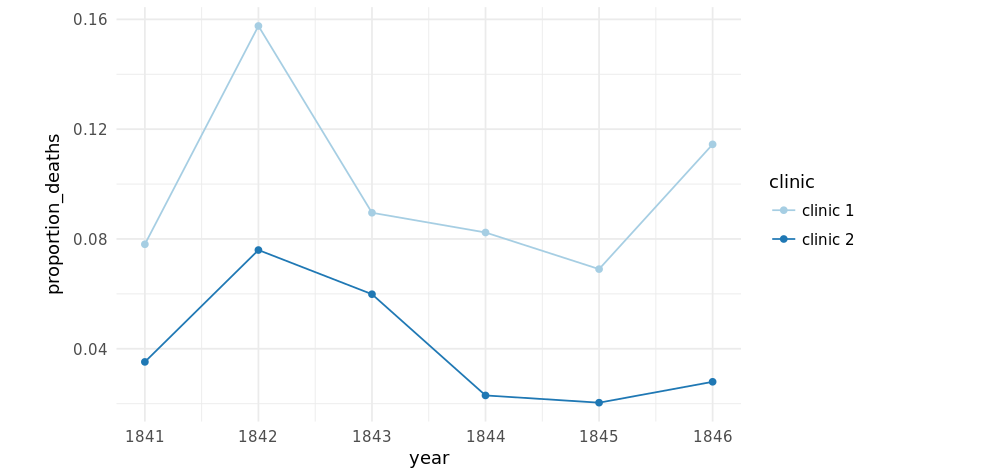## 4. The handwashing begins Why is the proportion of deaths constantly so much higher in Clinic 1? Semmelweis saw the same pattern and was puzzled and distressed. The only difference between the clinics was that many medical students served at Clinic 1, while mostly midwife students served at Clinic 2. While the midwives only tended to the women giving birth, the medical students also spent time in the autopsy rooms examining corpses. Semmelweis started to suspect that something on the corpses, spread from the hands of the medical students, caused childbed fever. So in a desperate attempt to stop the high mortality rates, he decreed: Wash your hands! This was an unorthodox and controversial request, nobody in Vienna knew about bacteria at this point in time. Let’s load in monthly data from Clinic 1 to see if the handwashing had any effect. # Read datasets/monthly_deaths.csv into monthly monthly &lt;- read_csv(&quot;datasets/monthly_deaths.csv&quot;) # Adding a new column with proportion of deaths per no. births monthly$proportion_deaths&lt;-monthly$deaths/monthly$births

# Print out the first rows in monthly

date births deaths proportion_deaths
1841-01-01 254 37 0.145669291
1841-02-01 239 18 0.075313808
1841-03-01 277 12 0.043321300
1841-04-01 255 4 0.015686275
1841-05-01 255 2 0.007843137
1841-06-01 200 10 0.050000000

## 5. The effect of handwashing

With the data loaded we can now look at the proportion of deaths over time. In the plot below we haven’t marked where obligatory handwashing started, but it reduced the proportion of deaths to such a degree that you should be able to spot it!

ggplot(data=monthly, aes(x=date, y=proportion_deaths)) +
geom_line() + geom_point()+
scale_color_brewer(palette="Paired")+
theme_minimal()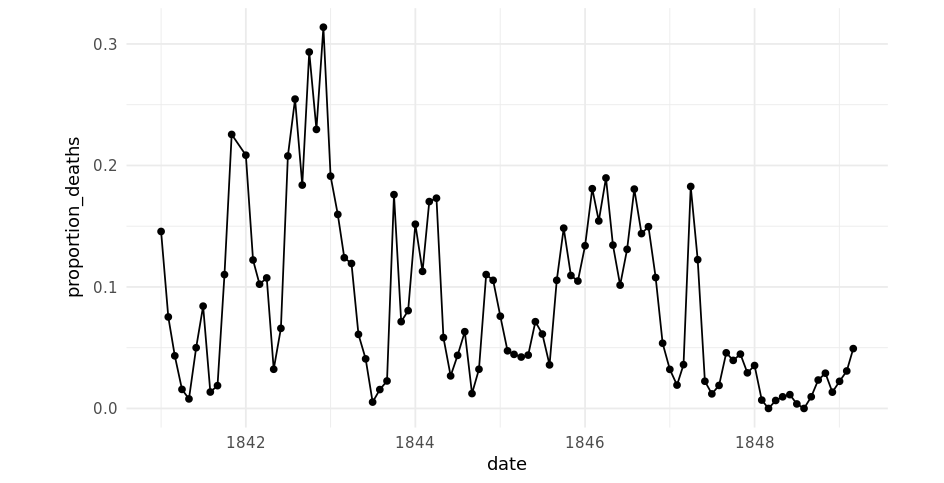## 6. The effect of handwashing highlighted¶

Starting from the summer of 1847 the proportion of deaths is drastically reduced and, yes, this was when Semmelweis made handwashing obligatory.

The effect of handwashing is made even more clear if we highlight this in the graph.

# From this date handwashing was made mandatory

handwashing_start = as.Date('1847-06-01')

# Add a TRUE/FALSE column to monthly called handwashing_started
monthly$handwashing_started=handwashing_start,TRUE,FALSE) # Plot monthly proportion of deaths before and after handwashing ggplot(data=monthly, aes(x=date, y=proportion_deaths, group=handwashing_started, color=handwashing_started)) + geom_line() + geom_point()+ scale_color_brewer(palette="Paired")+ theme_minimal()  ##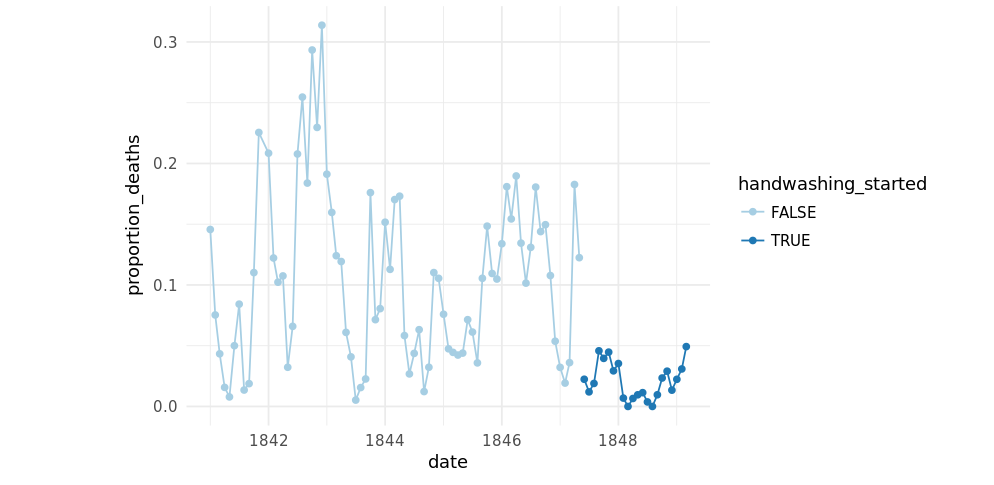## 7. More handwashing, fewer deaths? Again, the graph shows that handwashing had a huge effect. How much did it reduce the monthly proportion of deaths on average? # Calculating the mean proportion of deaths # before and after handwashing. monthly_summary % group_by(handwashing_started) %&gt;% summarise(mean_proportion_detahs=mean(proportion_deaths)) # Printing out the summary. monthly_summary  handwashing_started mean_proportion_detahs FALSE 0.10504998 TRUE 0.02109338 ## 8. A statistical analysis of Semmelweis handwashing data It reduced the proportion of deaths by around 8 percentage points! From 10% on average before handwashing to just 2% when handwashing was enforced (which is still a high number by modern standards). To get a feeling for the uncertainty around how much handwashing reduces mortalities we could look at a confidence interval (here calculated using a t-test). # Calculating a 95% Confidence intrerval using t.test test_result &lt;- t.test( proportion_deaths ~ handwashing_started, data = monthly) test_result  ## 9. The fate of Dr. Semmelweis That the doctors didn’t wash their hands increased the proportion of deaths by between 6.7 and 10 percentage points, according to a 95% confidence interval. All in all, it would seem that Semmelweis had solid evidence that handwashing was a simple but highly effective procedure that could save many lives. The tragedy is that, despite the evidence, Semmelweis’ theory — that childbed fever was caused by some “substance” (what we today know as bacteria) from autopsy room corpses — was ridiculed by contemporary scientists. The medical community largely rejected his discovery and in 1849 he was forced to leave the Vienna General Hospital for good. One reason for this was that statistics and statistical arguments were uncommon in medical science in the 1800s. Semmelweis only published his data as long tables of raw data, but he didn’t show any graphs nor confidence intervals. If he would have had access to the analysis we’ve just put together he might have been more successful in getting the Viennese doctors to wash their hands. / ## Export Data from Power BI into a file using R We usually import Data from file into Power BI, but exporting data from Power BI can be very handy when you want to create a custom visual using R. In fact it can be very cumbersome to code your visual directly into the Power BI script editor. Here are few reasons why you should opt for exporting your Power Bi dataset first and re-import it in R to create your visual. • Intellisense is not available in Power BI R script embedded • Does not highlight keywords in colour • Hard to debug & hard to code (you can’t print intermediate calculation) • Slower than Rstudio So unlike you’re a R master or you want to create a very simple visual it is definitely worth exporting your data into a file and then re-import it into R. You can then create your visual in Rstudio first and once you’re happy with it just copy and paste your code into the Power BI visual script. ### Export you data If you haven’t already installed the package (gdata) you’ll need to install it: #open an instance of R and type the command below install.packages("gdata");  Once the “gdata” package is installed, select the R visual script and drag into values the measures and columns you need.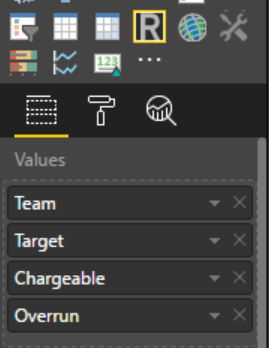In the R script editor type the following R code: require(gdata) write.table(trim(dataset), file="your filepath.txt", sep = "\t", row.names = FALSE)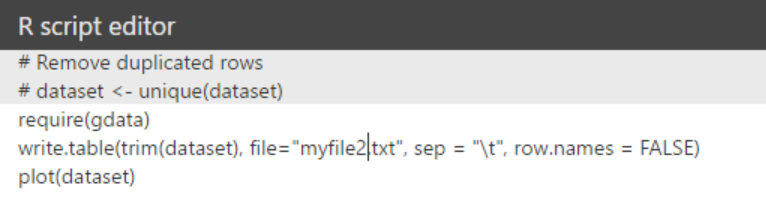You can add plot(dataset) like I did int the above screenshot to make sure there isn’t any errors in your script hence as long as you can see a plot whatever it is(line-plot, box-plot, correlation-plot) it means your export was successful or obviously you can just check if your file is present in your directory. Here is my output file: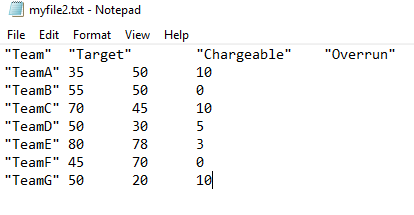### Re-import you Power BI dataset into R Now we can import our Power BI dataset into R as follows: dataset = read.table(file="myfile2.txt", sep = "\t",header = TRUE)  See the R output below: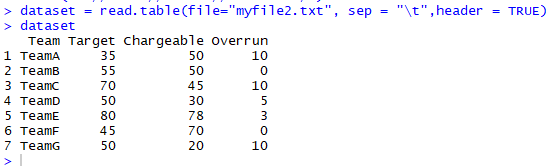You can now work with your dataset in Rstudio until you get your visual right and then you’ll just need to copy & paste your code into the Power Bi script.. / ## R – Import multiple CSV files and load them all together in a single dataframe ### List of all the filenames One approach I found really straight forward is just to create a list of all your filenames. You can also create a pattern to fetch your directory and returns all the matching files. In my example I need to read all the files starting with “FR”. setwd("H:/R Projetcs/Accidents") fileNames&lt;-Sys.glob(&quot;csv/FR*.csv&quot;) zonnesFiles&lt;- lapply(fileNames, read.csv)  The function lapply (equivalent of a loop) reads every single file presents in my list fileNames and store them into my variable zonnesFiles. The variable zonnesFiles is a list of data frames, I have to read 15 files so there's 15 different dataframes in the list. ### Merge all the files into a single data frame Once we have our list of dataframe we want to merge them in one single dataframe. As my files don’t have any headers I first need to make sure they all have the same column names, to do so I loop through my list of zonnesFiles and rename them. I then create a function “merge.all”, my function just call the base r “merge” function but I like to create my own so I don’t have to bother with parameter every time I need to call the function. Finally we just need to call our function for every single df in the zonnesFIles list. I use the Reduce function to successively merge each dataframe of my list. The Reduce function takes a binary function and a vector/list and successively applies the function to the list elements. And here is the code: #Rename column names of each df for(i in 1:length(zonnesFiles)){ colnames(zonnesFiles[[i]])&lt;-c(&quot;Longitude&quot;,&quot;Latitude&quot;,&quot;Type&quot;) } #Create a function to merge my df merge.all&lt;- function(x, y) { merge(x, y, all=TRUE, by=listCols) } #Lits of columns to merge on listCols&lt;-c(&quot;Longitude&quot;,&quot;Latitude&quot;,&quot;Type&quot;) #call the merge function zonnes&lt;- Reduce(merge.all, zonnesFiles)  / ## PowerBI – Dynamic Chart Title Unlike Qlikview, the chart titles in PowerBI can only be static. as you can only pass a static text in the title parameter.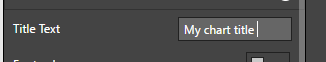However, there’s a way around it! The workaround I found is pretty simple you just need to fake a title by creating a measure which contains your title expression and drop this measure into a Card visual . Then by applying the same transparency and colours of your chart you just need to turn off the chart tile and put the Card visual on top of your chart. Here is the code for my title measure:  MyMeasureTitle = ("Total Cost of the Top " &amp; [TopN Value] &amp; " Depts VS all other Depts")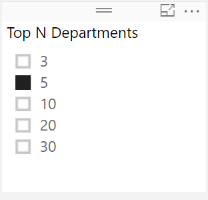So my title will interact with the above slicer dynamically however if no values are ticked off I still want a default value to be returned so here is the code for this (you might not need to implement it) TopN Value = IF ( HASONEVALUE ('TopN Filter'[TopN]) , VALUES ('TopN Filter'[TopN]) , 10 )  So after dropping your measure into a Card visual you’ve got your title ready!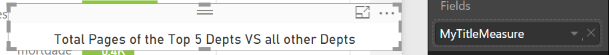And this how it looks when you place it right above your chart: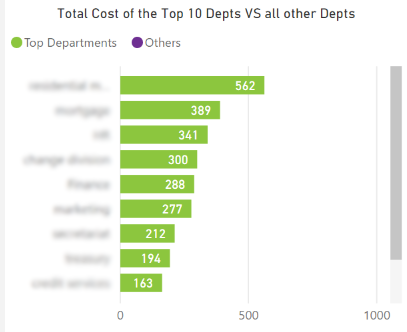Make sure your chart and the card have the same size and colour and by setting the right location x,y it will look like the embedded chart title. / ## For Loop vs Vectorization in R ### A brief comparison between for loop and vectorization in R A short post to illustrate how vectorization in R is much faster than using the common for loop. In this example I created two vectors a and b witch will take some random numbers. I’ll compute the sum of a and b using the for loop and the vectorization approach and then compare the execution time taken by both of the different methods. I’ll repeat this test 10 times with a different vector size, in the first test the vectors a and b contain only 10 elements but in the last test they contain 10 million elements. Here is the code for the loop version, when i=1 n=10 so we loop 10 times and when i=10 n=10,000,000 hence we loop 10 million times. ### For Loop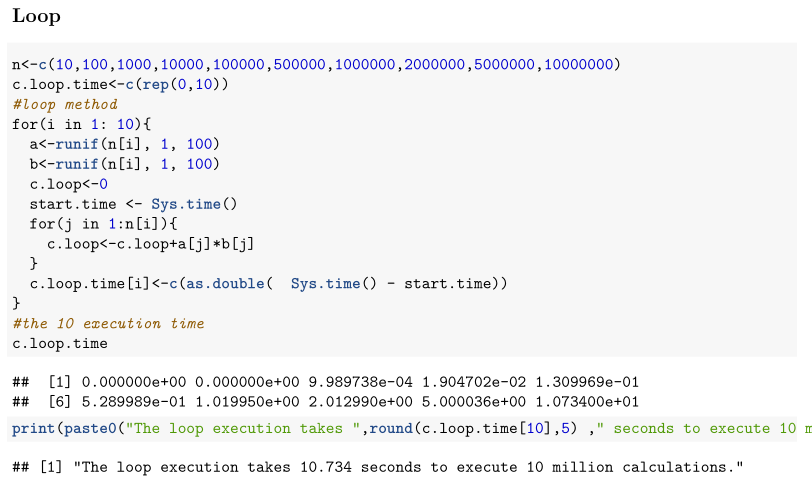I’ve stored the execution time taken for each test in the vector c.loop.time and I printed the last execution time when n=10 million. It took around 11 seconds to compute the sum of 10 millions values, let’s if we can do better with the vectorization method. ### Vectorization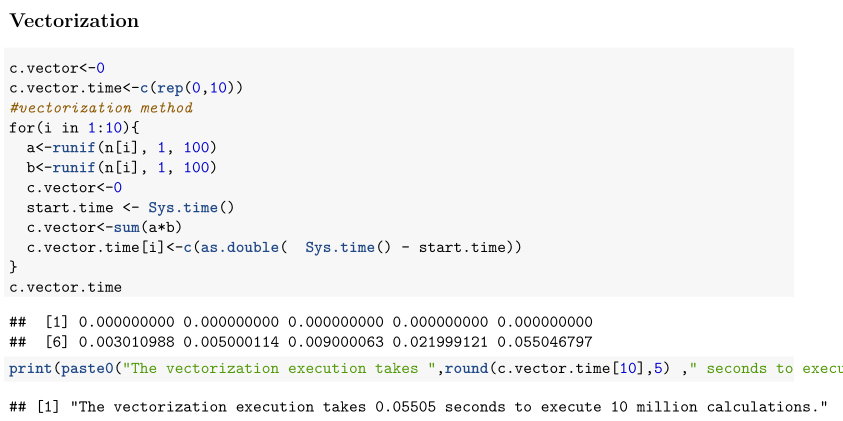With the vectorization method it took only around 0.05 seconds just five hundredths of a second, this is a two hundreds time faster than the for loop version! ### Result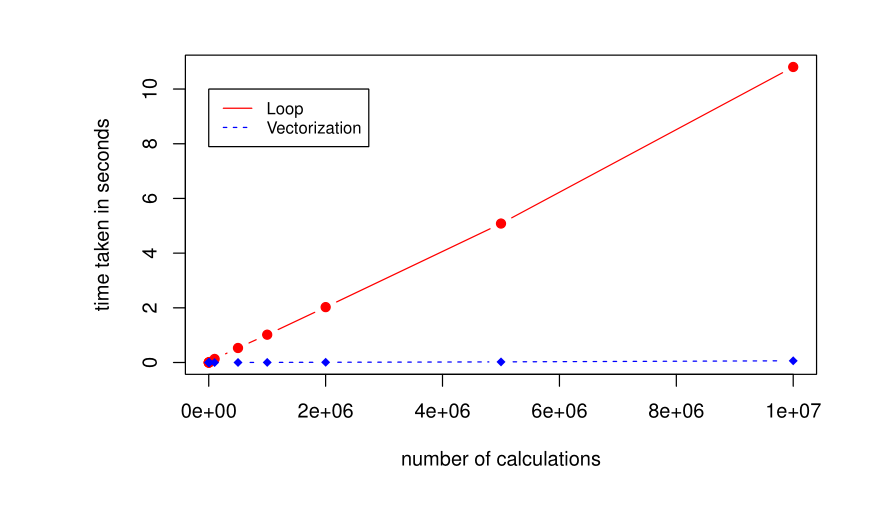This massive difference is mainly because in the for loop method we’re doing the computation at the R level resulting in many more function calls that all need to be interpreted and compiled (especially the variable affectation which occurs 10 million times). In the vectorization method the computation happens within the compiled code (C or Fortran I’m not too sure) and hence R has far less function to interpret and far fewer calls to compiled code. / ## Central Limit Theorem (example using R) The Central Limit Theorem is probably the most important theorem in statistics. The central limit theorem (CLT) states that given a sufficiently large sample size from a population with a finite level of variance, the mean of all samples from the same population will be approximately equal to the mean of the original population. Furthermore, the CLT states that as you increase the number of samples and the sample size the better the distribution of all of the sample means will approximate a normal distribution (a “bell curve“) even if the original variables themselves are not normally distributed. Let’s break down this with some examples using R: ### Original Population with a left skewed distribution Let’s generate our left skewed distribution in R. By using the rbeta function below I generated 10,000 random variables between 0 and 1 and I deliberately changed the shape parameter to have a distribution with a negative skewness. myRandomVariables<-rbeta(10000,5,2)*10The mean (µ) of the total population is 7.13 and the standard deviation (σ) is 1.61. We can see that the distribution has a tail longer on the left with some data that go up to 4 standard deviation away from the mean where as the data on the right don’t go beyond 2σ away from the mean. As we can see on the plot above the standard deviation (σ) allow us to see how far away from the mean each data are. A small σ means that the values in a statistical data set are close to the mean of the data set, on average, and a large σ means that the values in the data set are farther away from the mean, on average. AS the σ is 1.61 it mean that all the data between 5.52 (7.13-1.61) and 8.74 (7.13+1.61) are close the the mean (less than 1 σ). However the data less than 2.30 (7.13-3*1.61) are much more far from the mean at least 3σ. To better illustrate let’s see the same plot with the data scaled such as the mean is equal to 0 and the standard deviation is equal to 1. The formula to get the data normalised is (x-µ) / σ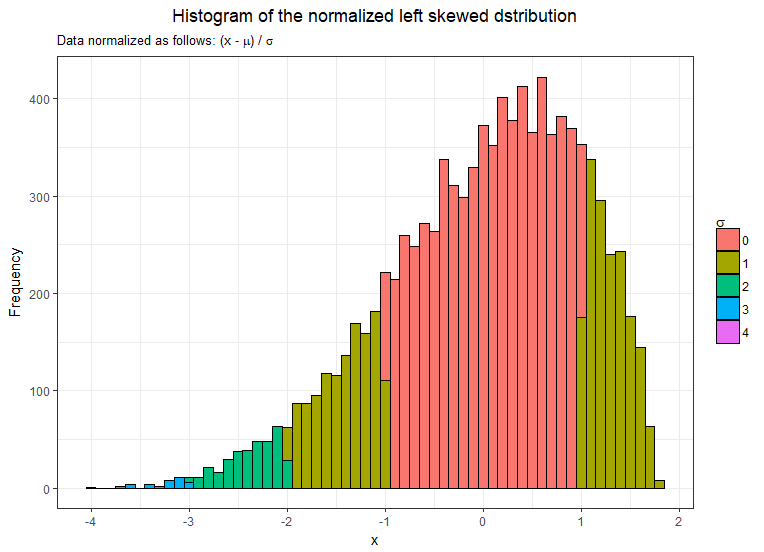The distribution still has exactly the same shape but it’s just make it easier to observe how the data are close or far from the mean. ### Using the Central Limit Theorem to Construct the Sampling Distribution So how can we use the CLT to construct the sampling distribution. We’ll use what we know about the population and our proposed sample size to sketch the theoretical sampling distribution. The CLT states that: • Shape of the sampling distribution: As long as our sample size is sufficiently large (>=30 is the most common but some textbook use 20 or 50) we should assume the distribution of the sample means to be approximately normal disregarding the shape of the original distribution. • The mean of the distribution (x̅): The mean of the sampling distribution should be equal to the mean of the original distribution. • The standard error of the distribution (σx): The standard deviation of the sample means can be estimated by dividing the standard deviation of the original population by the square root of the sample size. σx = σ/√n #### Let’s prove it then! I will first draw 100 mean samples from the original population with the minimum size recommended by the CLT 30. Here is the code to generate the sample means: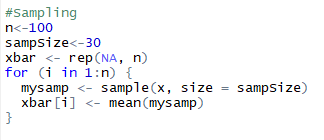So according to the CLT theorem the three following statements should be true: 1. The mean of our sample means distribution should be around 7.13 2. The shape of the sample distribution should be approximately normal 3. Standard error (σx = σ/√nshould be equal to 0.29 (1.61/√30)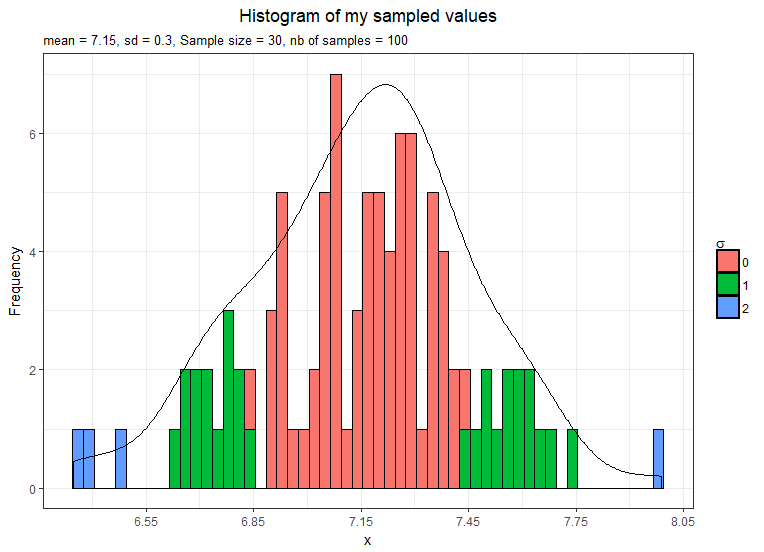1. The mean is 7.15 hence nearly 7.13 2. The shape is approximately normal still a little bit left-skewed 3. The standard error is 0.3 hence nearly 0.29 The CLT also states that as you increase the number of samples the better the distribution of all of the sample means will approximate a normal distribution. #### Let’s draw more samples. Now I take 1,000 samples means and plot them.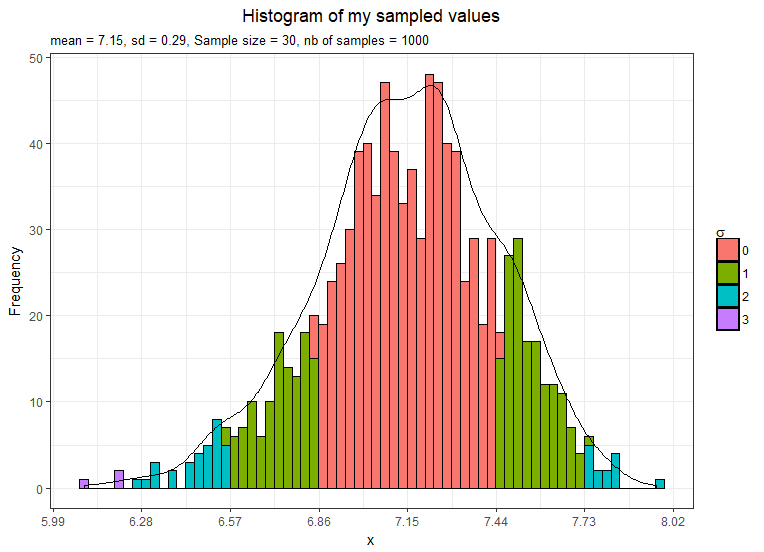1. The mean is still 7.15 and not exactly 7.13 2. The shape is approximately normal but still a little bit left-skewed 3. The standard error is equal to 0.29 as estimated by the CLT theorem #### Let’s take even more sample means. This time I take 10,000 samples means and plot them.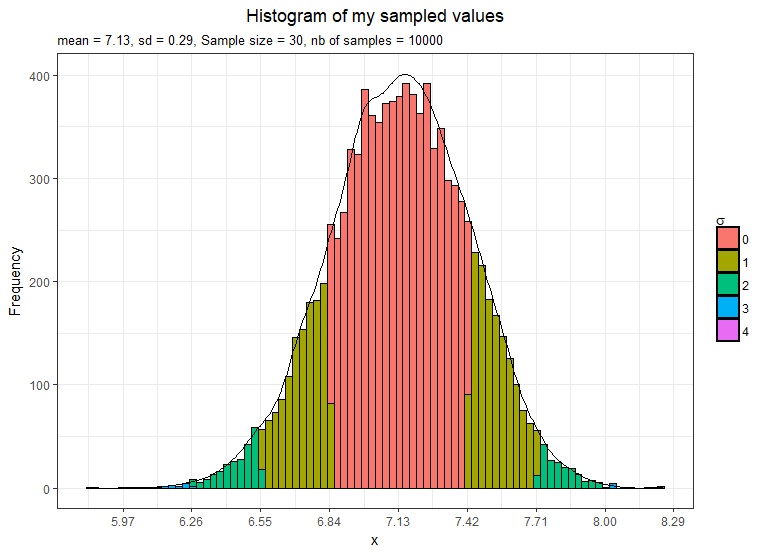1. The mean is now exactly 7.13 2. The distribution shape is definitely normal 3. The standard error is equal to 0.29 as estimated by the CLT theorem Just for the fun let’s do another example and this time with a different sample size to see if we get the standard error right. So using the CLT theorem the σx should be 0.11 (1.61/√200)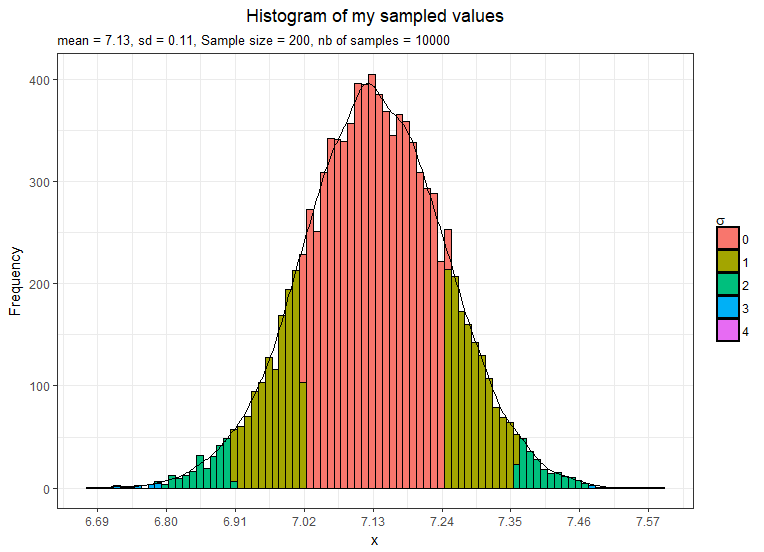We have increased each sample size to 200 instead of 30 in the previous examples hence the variance in the sample means distribution has decreased and we now have a standard error smaller. This confirms that as we increase the sample size the distribution becomes more normal and also the curve becomes taller and narrower. ### Summary The CLT confirms the intuitive notion that as we take more samples or as we increase the sample size , the distribution of the sample means will begin to approximate a normal distribution even if the original variables themselves are not normally distributed, with the mean equal to the mean of the original population (x̅=µ) and the standard deviation of the sampling distribution equal to the standard deviation of original the population divided by the square root of the sample size (σx = σ/√n). In the next post I’ll talk more in depth about the CLT and we’ll see how and where we can use the CLT. / ## Coursera Data Science Specialization Review# “Ask the right questions, manipulate data sets, and create visualizations to communicate results.” “This Specialization covers the concepts and tools you’ll need throughout the entire data science pipeline, from asking the right kinds of questions to making inferences and publishing results. In the final Capstone Project, you’ll apply the skills learned by building a data product using real-world data. At completion, students will have a portfolio demonstrating their mastery of the material.” The JHU Data Science Specialization is one of the earliest MOOC that has been available online with the Machine Leaning from Andrew NG and The Analytic Edge on EDX. The data science specialization consists of 9 courses and one final capstone project. Each course is a combination of video with graded quizzes, and peer graded projects. The list of the courses is as follows: Course 1: The Data Scientist’s Toolbox Course 2: R Programming Course 3: Getting and Cleaning Data Course 4: Exploratory Data Analysis Course 5: Reproducible Research Course 6: Statistical Inference Course 7: Regression Models Course 8: Practical Machine Learning Course 9: Developing Data Products Course 10: Capstone project So far I’ve completed the 9 courses and I’m still working on the final capstone project. Here is my overall review about the data science specialization: ## Strengths • The first courses are very easy and you don’t need a data science or a heavy math background to complete the different courses, however, having a descent programming skills and good statistics background will be an advantage. • The specialization uses R, Github and Rpubs all those tools are compeltely free. R is nowadays one of the most popular statistical language with Python and SAS (very expensive). Also there’s a really big community supporting R. • The specialization covers a broad of different topics such as R programming, statistics inference, exploratory data analysis, reproducible research and machine learning. • Each course contains at least one project and this is where you get to learn the most. I always found that the moment I learn the most is actually when I take a test even if I fail or when I work on a real project. ## Weaknesses • Because the specialization is intended to a public with no heavy math background and no previous exposure to R the courses were a bit slow at the beginning. • In the other hand if you’re not familiar with statistical inference you might find yourself struggling to understand some concepts as the professor Brian Caffo tends to go a bit fast on some essential notion of statistics. • The price, 37£/ month so the quicker you finish the cheaper it costs, the price is still affordable but the first courses are definitely not worth it as you can just dowload the Siwrl package in R and follow the tutorial however if you want the final certificate you do need to complete all the 9 courses and the final project. You can still audit the courses free, you’ll have access to all the videos but you won’t have access to the project homework which is the best part of this MOOC. • Finally, the main drawback of this MOOC is the peer grade assignment some students take it very seriously and review your work properly and give a good feedback where as some students don’t even bother reviewing your work. ## Brief overview of each course ### Course 1: The Data Scientist’s Toolbox “In this course you will get an introduction to the main tools and ideas in the data scientist’s toolbox. The course gives an overview of the data, questions, and tools that data analysts and data scientists work with. There are two components to this course. The first is a conceptual introduction to the ideas behind turning data into actionable knowledge. The second is a practical introduction to the tools that will be used in the program like version control, markdown, git, GitHub, R, and RStudio.” #### Review This course is a big joke they shouldn’t charge for it, if you know how to use github and install R you’re done… ### Course 2: R Programming In this course you will learn how to program in R and how to use R for effective data analysis. You will learn how to install and configure software necessary for a statistical programming environment and describe generic programming language concepts as they are implemented in a high-level statistical language. The course covers practical issues in statistical computing which includes programming in R, reading data into R, accessing R packages, writing R functions, debugging, profiling R code, and organizing and commenting R code. Topics in statistical data analysis will provide working examples. #### Review If you already have a programming background and you understand the concept of vector, matrix and data.frame manipulation this course will be really easy. However if you’re not familiar with programming or don’t know R at all this course is definitely worth it. ### Course 3: Getting and Cleaning Data Before you can work with data you have to get some. This course will cover the basic ways that data can be obtained. The course will cover obtaining data from the web, from APIs, from databases and from colleagues in various formats. It will also cover the basics of data cleaning and how to make data “tidy”. Tidy data dramatically speed downstream data analysis tasks. The course will also cover the components of a complete data set including raw data, processing instructions, codebooks, and processed data. The course will cover the basics needed for collecting, cleaning, and sharing data. #### Review Well this course teaches the essential knowledge of reading and cleansing data. In this course you get exposed to the dplyr package which is I think one the most popular and important package to master. However when ever you want to read a specific file or do a specific string manipulation in R you just google it and you find the answer so no need to watch dozens and dozens video for it. Not worth it. ### Course 4: Exploratory Data Analysis This course covers the essential exploratory techniques for summarizing data. These techniques are typically applied before formal modeling commences and can help inform the development of more complex statistical models. Exploratory techniques are also important for eliminating or sharpening potential hypotheses about the world that can be addressed by the data. We will cover in detail the plotting systems in R as well as some of the basic principles of constructing data graphics. We will also cover some of the common multivariate statistical techniques used to visualize high-dimensional data. #### Review I really liked this course and it’s definitely worth it. First ggplot is a must in R, plotting data is where to start in Data Science if you want to analyse data and start making assumption ggplot is your guy. In addition to ggplot you’ll get exposed to the K-means algorithm (clustering algorithm) and the PCA (dimensions reduction algorithm) and Brian skips all the math. You’ll see PCA again the course 8 “Machine Learning” but still the course will skip the core math and will not go deep enough to really understand its concept. ### Course 5: Reproducible Research This course focuses on the concepts and tools behind reporting modern data analyses in a reproducible manner. Reproducible research is the idea that data analyses, and more generally, scientific claims, are published with their data and software code so that others may verify the findings and build upon them. The need for reproducibility is increasing dramatically as data analyses become more complex, involving larger datasets and more sophisticated computations. Reproducibility allows for people to focus on the actual content of a data analysis, rather than on superficial details reported in a written summary. In addition, reproducibility makes an analysis more useful to others because the data and code that actually conducted the analysis are available. This course will focus on literate statistical analysis tools which allow one to publish data analyses in a single document that allows others to easily execute the same analysis to obtain the same results. #### Review The course teaches how to use Rmarkdown and other tool/languages to write and publish documents which contain data analysis. I found Rmarkdown really handful and if you want to share your work with the comunity on Rstudio, Rpubs or Kaggle, Rmarkdown is a must. So I found this course quite useful as well. ### Course 6: Statistical Inference Statistical inference is the process of drawing conclusions about populations or scientific truths from data. There are many modes of performing inference including statistical modeling, data oriented strategies and explicit use of designs and randomization in analyses. Furthermore, there are broad theories (frequentists, Bayesian, likelihood, design based, …) and numerous complexities (missing data, observed and unobserved confounding, biases) for performing inference. A practitioner can often be left in a debilitating maze of techniques, philosophies and nuance. This course presents the fundamentals of inference in a practical approach for getting things done. After taking this course, students will understand the broad directions of statistical inference and use this information for making informed choices in analyzing data. #### Review This course is a big disappointment! Statistical inference are really fundamental in Data Science, JH University tried to fill this course in four weeks and as a result the course is completely botched. That’s a big shame that’s they try to pack this course in four weeks they could heave easily split this course in two course and get rid of data product or data science toolbox instead. Luckily I have a degree with minor in Statistics so I didn’t struggle with the exams however if you’re not familiar with statistical inference I would definitely recommend you to study with another material. (Foundations of Data Analysis part 1 & 2 on EDX could be a good one as it’s using R as well and it’s completely free!) ### Course 7: Regression Models Linear models, as their name implies, relates an outcome to a set of predictors of interest using linear assumptions. Regression models, a subset of linear models, are the most important statistical analysis tool in a data scientist’s toolkit. This course covers regression analysis, least squares and inference using regression models. Special cases of the regression model, ANOVA and ANCOVA will be covered as well. Analysis of residuals and variability will be investigated. The course will cover modern thinking on model selection and novel uses of regression models including scatterplot smoothing. #### Review Again there’s no chance you can get a solid grasp of regression model with this course. Too short the coverage of regression model is far from complete. it tells you how to run a linear or log regression in R and tell only a little bit about the interpretation and optimization of a model. However this time there were few optional videos will all the math involved behind the algorithm I think they should add these optional video for every single algorithms for the people who would like to go deeper or just enjoy the magic of math. ### Course 8: Practical Machine Learning One of the most common tasks performed by data scientists and data analysts are prediction and machine learning. This course will cover the basic components of building and applying prediction functions with an emphasis on practical applications. The course will provide basic grounding in concepts such as training and tests sets, overfitting, and error rates. The course will also introduce a range of model based and algorithmic machine learning methods including regression, classification trees, Naive Bayes, and random forests. The course will cover the complete process of building prediction functions including data collection, feature creation, algorithms, and evaluation. #### Review This one was my favourite! In this course you will use the caret package another must. The caret package is really useful for data spliting, pre-processing, feature selection and model tuning. This course was mainly taught by Roger D. Peng and he used a very practical approach that I really liked. This course covers different areas of machine learning and gives a foretaste of further area of study. Definitely worth it. ### Course 9: Developing Data Products A data product is the production output from a statistical analysis. Data products automate complex analysis tasks or use technology to expand the utility of a data informed model, algorithm or inference. This course covers the basics of creating data products using Shiny, R packages, and interactive graphics. The course will focus on the statistical fundamentals of creating a data product that can be used to tell a story about data to a mass audience. #### Review Well it just repeats what was said in the reproducible research and for the project you have to realize an interactive dashboard using shiny and plotty. Well I’m a BI consultant so I like doing dashboard either with SSRS, PowerBI, Qlikview, Tableau but SHINY no more please!!! It took me several hours to do a horrible interactive dashboard instead of 2 minutes with a BI software. OK, I’m probably biased since I work in BI with not free tools. I think Shiny is still good for an internal usage or in small scale or maybe for very specific dashboard that cannot be done with normal BI tools… ### Course 10: Capstone project The capstone project class will allow students to create a usable/public data product that can be used to show your skills to potential employers. Projects will be drawn from real-world problems and will be conducted with industry, government, and academic partners. #### Review Again a big joke! You spent nearly 6 months learning different statistic method so you’re expected to work on a project that will combine all the different method you learnt. But no!! The project is about Natural Language Processing and there is actually no courses at all on this subject. NLP is a very challenging and interesting topic but the fact the final project is actually not related on the 9 previous courses is really frustrating. Anyway at least it’s still an interesting challenge and it’ll really help you to develop your solving problem skills and expand your knowledge about NLP. I haven’t finished the Capstone project yet I actually missed the deadline and so far my laptop wasn’t powerful enough to run the different algorithms I’ve implemented. (I’ve got 8 gb RAM). Here is an introduction of the work I’ve done for the final capstone project Exploratory analysis of SwiftKey dataset ## Summary The courses are mainly focused on teaching R and addressing some high level aspects of doing data science. I don’t think these courses are intended for beginner in programming and ML especially the capstone project and inferential statistics course. Also these courses are not good at all to get a good understating of statistics and to learn the different aspects of ML in detail. The best part of these courses is that you’ll learn R throughout the whole specialization so if you don’t know R already and want to get exposed to ML in the meantime this MOOC might be right for you. The Explanotary analysis and Machine Learning courses have really good content so if you already know R & R Markdown I’d definitely recommend to take those two courses and then skip the rest. Finally if you haven’t been exposed to R and statistics before I’d highly recommend to learn the basic of R with the swirl package and build up your statistics knowledge with Fondation Data Analysis part 1&2 on EDX. Linear algebra is not essential for these courses but it’ll help you to understand the more advanced concepts and math behind the different algorithms present in the Regression models course, and the PCA course and will also become essential if you want to delve into ML / ## Human Resources Data Analytics Using predictive analytics to predict the leavers. The dataset contains the different variables below: • Employee satisfaction level • Last evaluation • Number of projects • Average monthly hours • Time spent at the company • Whether they have had a work accident • Whether they have had a promotion in the last 5 years • Department • Salary • Whether the employee has left *This dataset is simulated Download dataset By using the summary function we can obtain the descriptive statistic information of our dataset: Data preparation: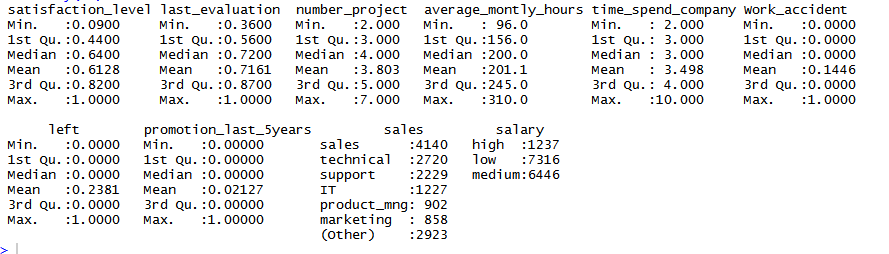Followed by the str function which returns data types of our variables: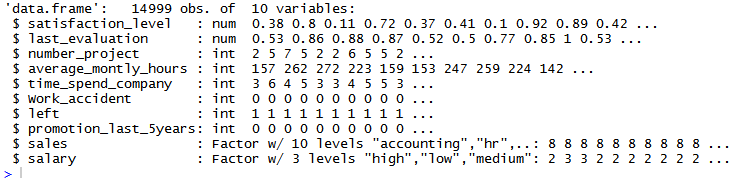Looking at my data I noticed that some variables are int type but can potentially be a factor type: Using the Unique function I can clearly identified all the factor variables such as work_accident, left, promotion_last_5years…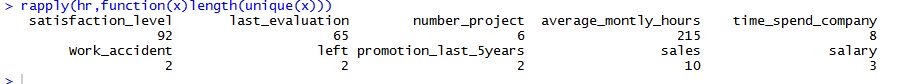To convert a data to a factor type I use the function as.factor(): eg with the variable left: hr$left<-as.factor(hr$left) I just double check that my variable is now a factor type: str(hr$left) –> Factor w/ 2 levels “0”,”1″:

Descriptive statistics:

Once our data are well cleaned and tidied up I can plot some charts to get some information about the data.

I first want to look at the distribution of each variable alone and then I’ll compare two variable with each other so I can figure out whether our data are correlated or not.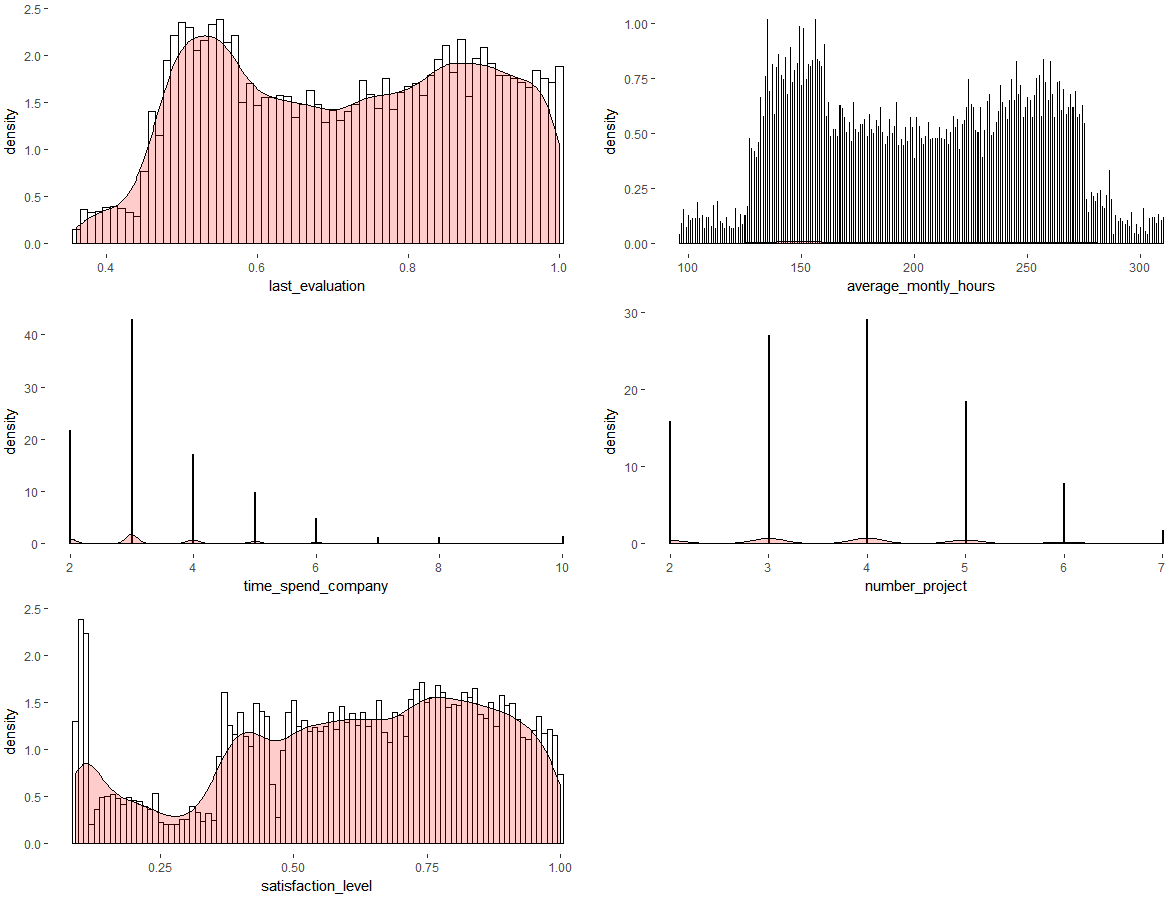The satisfaction_level variable looks like a multimodal distribution as well as last_evaluation and average_monthly_hour.

The first thought that comes to my mind hen I look at the satisfaction_level distribution is that the left peak is very likely to contains the leavers.

Density comparison:

I will no compare the density for different variables:

First thing I want to analyse is satisfaction_level against the variable left.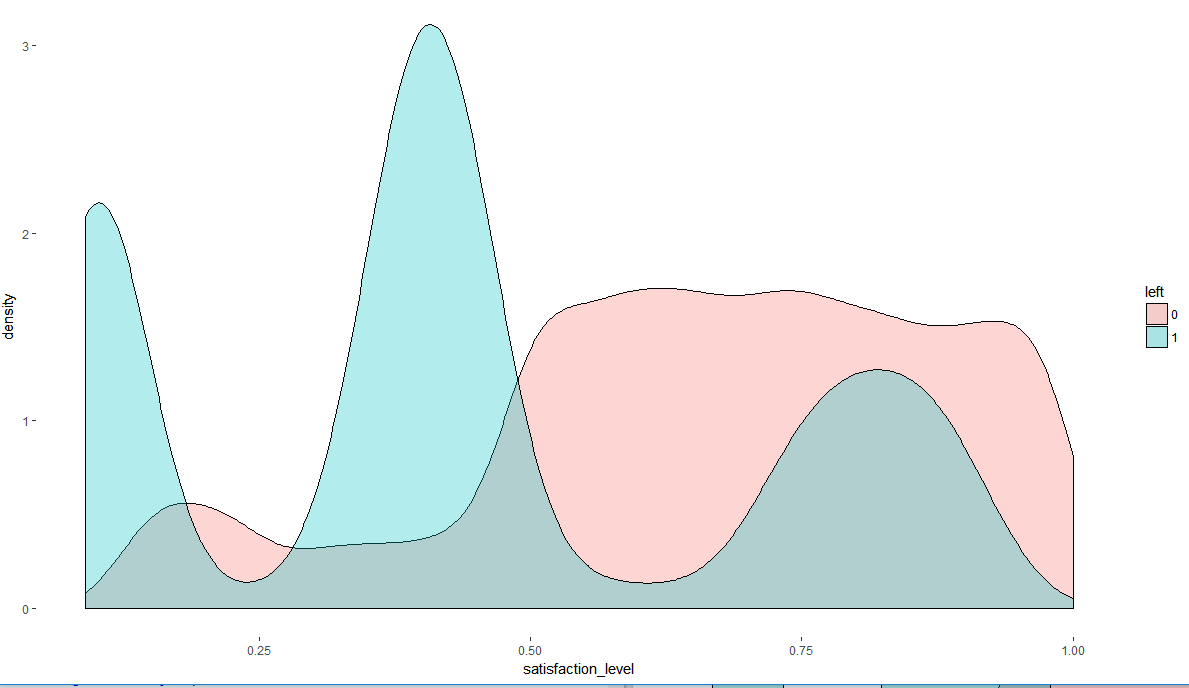This chart shows satisfaction level density for the variable left. We can clearly see that employees poorly satisfied are more likely to leave than highly satisfied ones.

Now I wonder if satisfaction_level is related to the salary: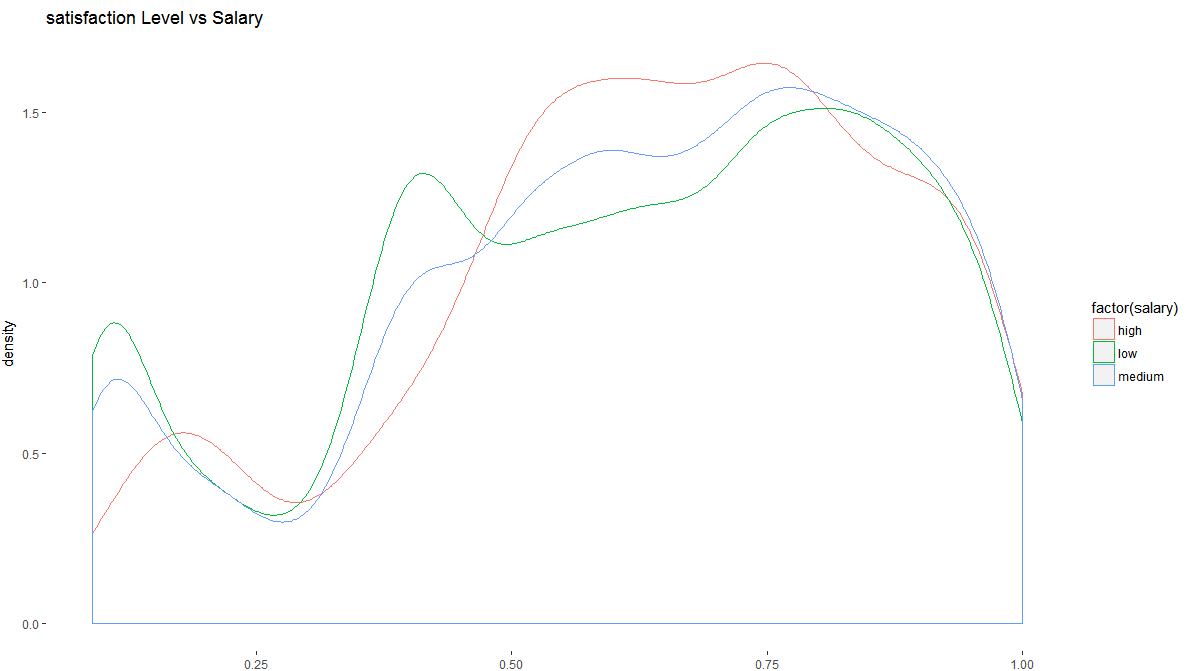Again we can observe a small peak on the left so we can tell that the salary has an impact on the satisfaction_level however this impact is not very significant which implies that there should be other variables correlated to the satisfaction_level.

Let’s compare couple of other variables against left: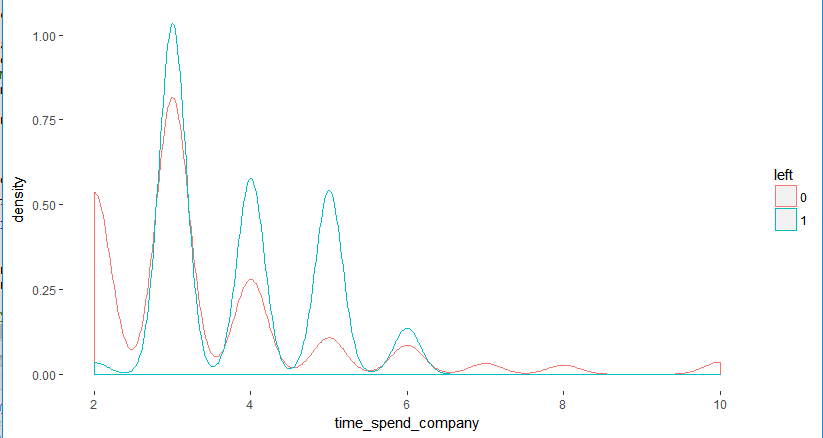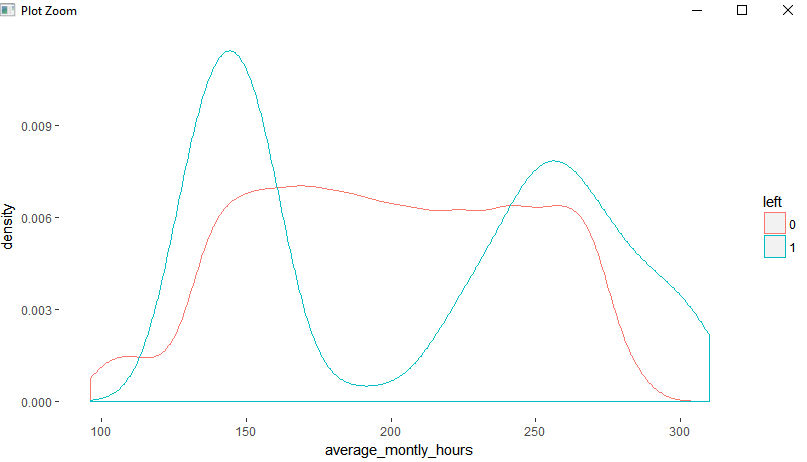Time_spend_company and average_monthly_hours seem also to have a little impact on the variable left.

SO far I have compared only continuous variables with discrete variables.

I am now interesting to compare the salary variable (low, medium, high) with the variable left (0 or 1).

One way to do that is by using a contingency table which returns the number of leavers/ non leavers for each salary category and then figure out if the variables are distributed uniformly.

The proportion table allows to get the percentage of leavers for each salary category instead of the number which make it easier to analyse.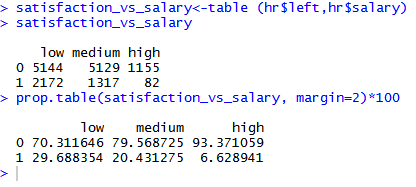And indeed the variables “left” and “salary” are clearly uniformly distributed.

The percentage of leavers among “high salary” category is only 6.6% while the proportion for the “low salary” is 29.6%.

We can also visualise this result by plotting factor left on the x  abscisse and get a line for each category salary.

By using a threshold of 0.5 we can also see that density of leavers is much bigger on the right of vertical line and opposite for the high salary which obviously implies that employees with lower salary are more likely to leave than employees with huger salary.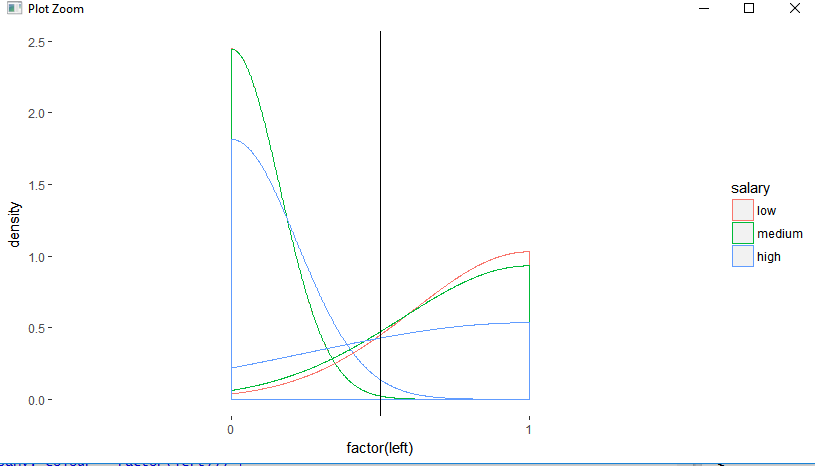Correlation and conclusion before further analysis:

OK, so far we have built quite a lot of charts and we can already predict an employee with a low salary, low satisfaction_level and who spend a lot of time in the company is very likely to be a leaver.

However our dataset is simulated and contains only few variables, usually, datasets are much bigger and contain a lot of columns so plotting every single variable with with one another to find a correlation will be too long.

One way to get a quick picture of all the correlation among numeric variables is to use the function cor():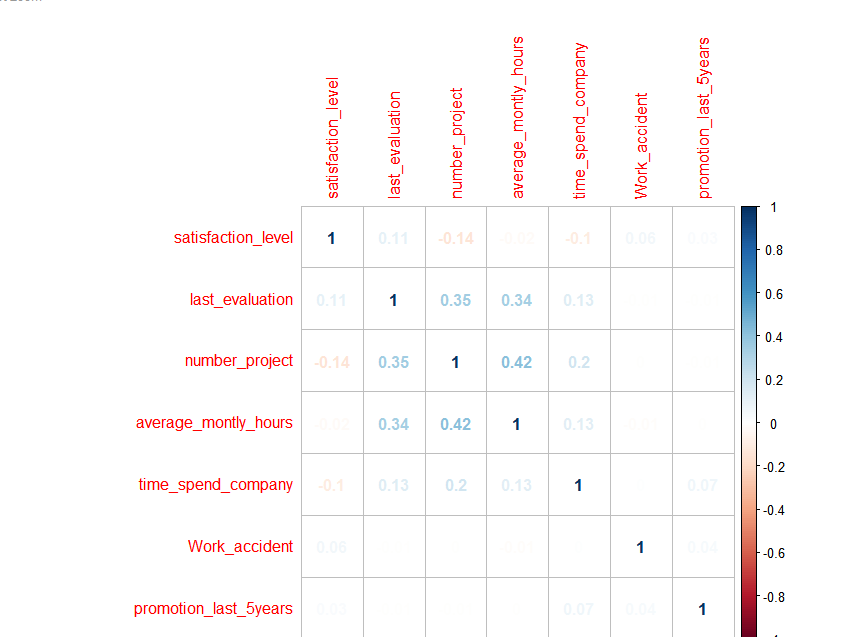Unfortunately, the cor() function does not produce tests of significance, also, this coefficient tells only about the linear relation between these variables and these variables are not linearly correlated

Data splitting: training 70%, testing 30%

In order to test the accuracy of our models we have to create a training subset which we will use to build our models and to create a testing subset which we will use to test the accuracy of our models.

By using the sample split function we can split our dataset into two subset.

By passing the independent variable “left” the split function will also make sure to keep the same proportion of leavers in both subset.

I just used a continence table on our two subsets to make sure we do have the same proportion of leavers, which indeed are still equal.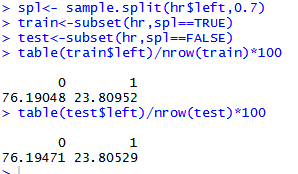Let’s build our predictive models

I will implement couple of different models and then compare their accuracy to find out which model model is the most accurate.

The different model that I will build are:

• Logistic regression
• Classification tree (CART)  (with different parameters)
• Minimum buckets = 100
• Minimum buckets = 50
• Minimum buckets =25
• Cross Validation for the CART model
• Random Forest

Logistic regression
modelglm<-glm(left~.,data=train,family=”binomial”)
test$prediction.glm<-predict(modelglm,type=”response”,newdata=test) summary(modelglm)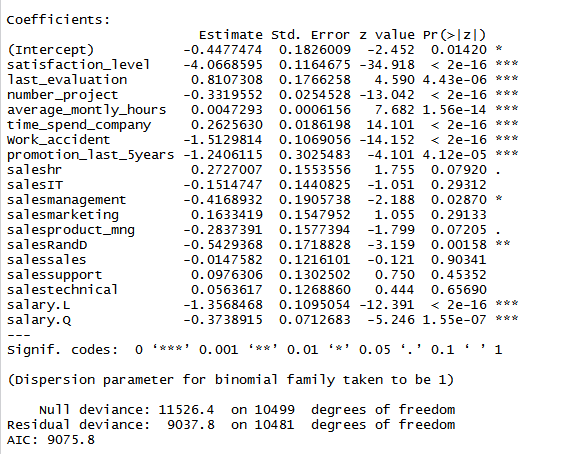Here the summary result of the regression logistic model, to be honest this is the first time I have ever seen a model with such significant variables. Satisfaction_level, number_project, time_spend_company, salary and work_accident are really significant they have a p-value equal to less than 10^-16 but remember that the dataset is simulated so this is not too surprising. As all the variables are significant I will keep all of them in model but I am sure I could easily removed few variables from this model as I suspect some multicollinearity between certain variables. The Area Under the Curve of my model is quite good as 0.826 is close to 1. The ROC curve is a way to evaluate the performance of a classifier using the specificity and sensitivity, the AUC has its pros and cons but still widely used. #Hopefully I will write a post specially for the AUC and the other ways to compare different classifiers.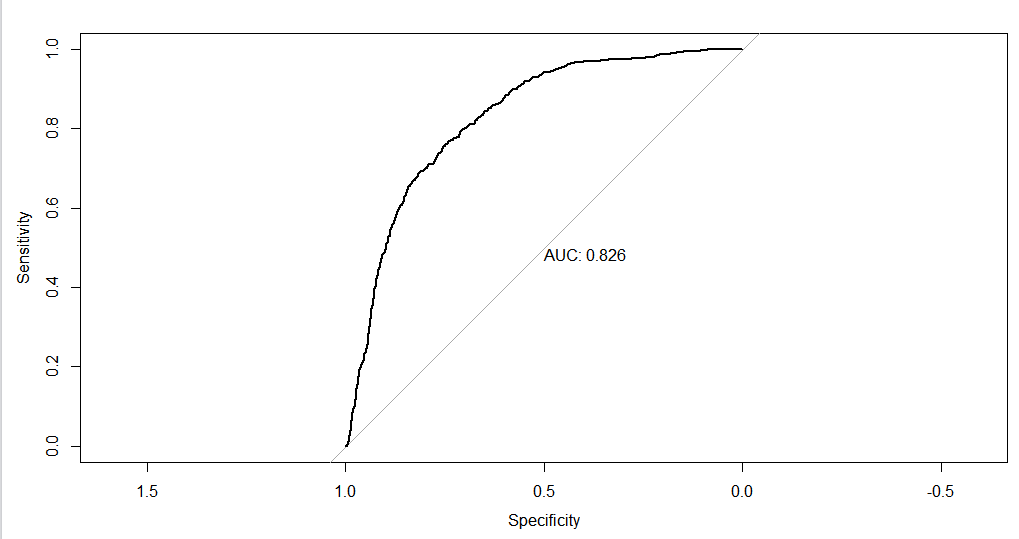Decision Tree (Model CART) Now I will build three different trees one with a minimum bucket/leaf of 100 then 50 then 25. • CART min bucket=100: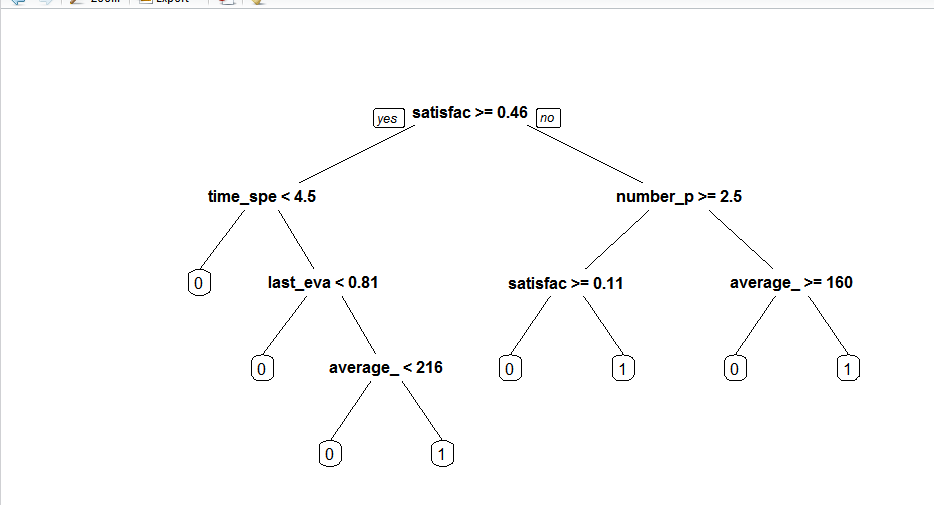I like using trees to demonstrate and explain the relationship between the data because it does not require any math skill do be understood. Obviously the math behind it is harder than a linear regression or a K-means algorithm but the result given given by a decision tree is very easy tor read. I this tree for example an employee with a degree of satisfaction >= 0.46 and number_project >= 2.5 and average_monthly_hours >=160 will be predicted as a leaver. • CART min bucket=50: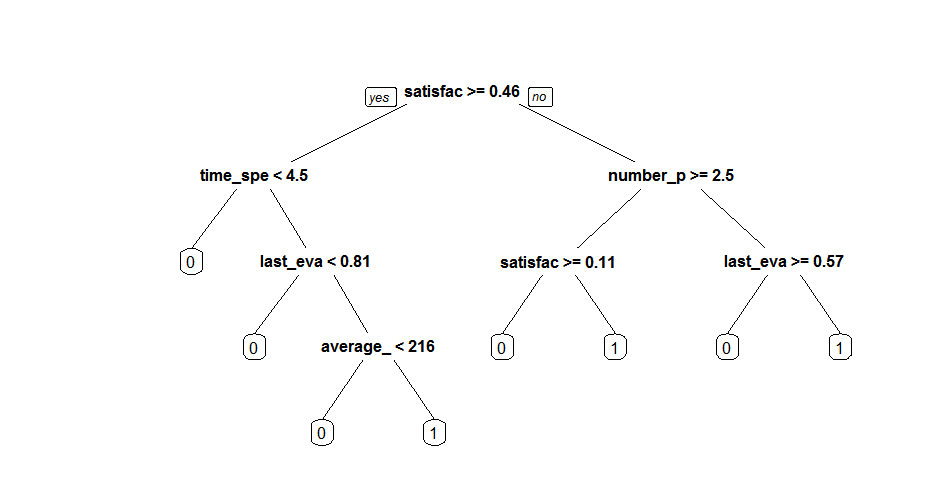• CART min bucket=25: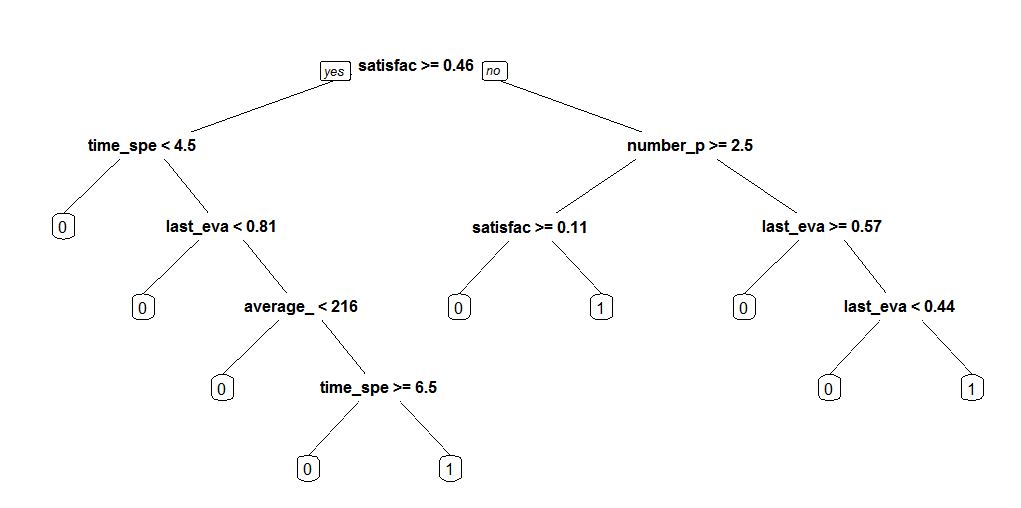More we decrease the number of minimum bucket in our model more the tree will get bigger. It’s not always easy to set the minimum bucket of our tree as we want to avoid over fitting or under fitting our model. So far I have built three classification tree models one regression logistic and I’ll test those models later against my test subset. Cross Validation K-fold cross validation consist in splitting our dataset into k subset (10 in our example) and the method is repeated K-times. I will talk more about k-fold CV in another post but in summarise k-fold CV is very useful for detecting and preventing over-fitting the data especially when the dataset is small. Each time one of the subsets is used all the other subsets are put together to form the training set. Every single observation will be in the testing set exactly once and k-1 times in the training so the variance will be averaged over the k different partitions so the variance will be much lower than a single hold-out set estimator. Random Forest / ## Populating a Time Dimension A ready-made script that I have modified to create and populate a Kimball Time dimension. This script will create a time dimension and populate it with different levels of granularity: second, minute, hour. --Create the time dim table CREATE TABLE [dbo].[DimTime]( [TimeKey] [int] NOT NULL, [TimeAltKey] [int] NOT NULL, [Time] [varchar](8) NOT NULL, [TimeMinutes] [varchar](5) NULL, [TimeHours] [varchar](2) NULL, [HourNumber] [tinyint] NOT NULL, [MinuteNumber] [tinyint] NOT NULL, [SecondNumber] [tinyint] NOT NULL, [TimeInSecond] [int] NOT NULL, CONSTRAINT [PK_DimTime] PRIMARY KEY CLUSTERED ( [TimeKey] ASC ) ) --Script to populate the time dimension CREATE PROCEDURE [dbo].[p_InsertDimTime] as BEGIN --Specify Total Number of Hours You need to fill in Time Dimension DECLARE @Size INTEGER --iF @Size=32 THEN This will Fill values Upto 32:59 hr in Time Dimension Set @Size=23 DECLARE @hour INTEGER DECLARE @minute INTEGER DECLARE @second INTEGER DECLARE @TimeKey INTEGER DECLARE @TimeAltKey INTEGER DECLARE @TimeInSeconds INTEGER DECLARE @Time varchar(25) DECLARE @hourTemp varchar(4) DECLARE @minTemp varchar(4) DECLARE @secTemp varchar(4) SET @hour = 0 SET @minute = 0 SET @second = 0 SET @TimeKey = 0 SET @TimeAltKey = 0 WHILE(@hour<= @Size ) BEGIN if (@hour <10 ) begin set @hourTemp = '0' + cast( @hour as varchar(10)) end else begin set @hourTemp = @hour end WHILE(@minute <= 59) BEGIN WHILE(@second <= 59) BEGIN set @TimeAltKey = @hour *10000 +@minute*100 +@second set @TimeInSeconds =@hour * 3600 + @minute *60 +@second If @minute <10 begin set @minTemp = '0' + cast ( @minute as varchar(10) ) end else begin set @minTemp = @minute end if @second Continue reading "Populating a Time Dimension" / ## Implement Linear Regression in R (single variable) Linear regression is probably one of the most well known and used algorithms in machine learning. In this post, I will discuss about how to implement linear regression step by step in R. Let’s first create our dataset in R that contains only one variable “x1” and the variable that we want to predict “y”. #Linear regression single variable data <- data.frame(x1=c(0, 1, 1), y = c(2, 2, 8)) #ScatterPlot plot(data, xlab=’x1′, ylab=’y’,xlim=c(-3,3), ylim=c(0,10))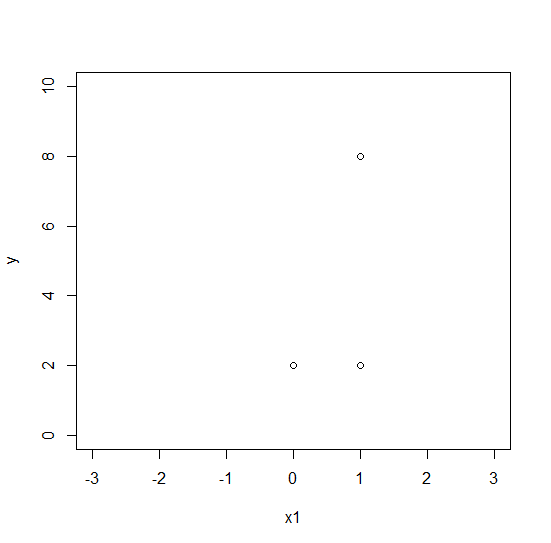We now have three points with coordinates: (0;2),(1;2),(1;8) and we want to dar the best fit line that will best represents our data on a scatter plot. In the part 1 I will implement the different calculation step to get the best fine using some linear algebra, however, in R we don’t need to do the math as there’s already a bult-in function called “lm” which computes the linear regression calculation. So, if you just want to use the linear regression function straight away and don’t go through the different step to implement a linear model you can skip the part 1 and go to the part 2. I’d still recommand to undertsand how the algorithm works than just using it. Part 1: Linear regression (with linear algebra calculation) In order to find the best fit line that minimizes the sum of the square differences between the left and right sides we’ll compute the normal equation:#Output vector y y = c(2, 2, 8) #Input vector x1 x1=c(0, 1, 1) #Intercept vector (it is simply the value at which the fitted line crosses the y-axis) x0<-rep(1,nrow(y)) #Let’s create my Y matrix Y <- as.matrix(data$y)

#Let’s create ny X matrix

X <- as.matrix(cbind(x0,data\$x1))

#Let’s compute the normal equation

beta = solve(t(X) %*% X) %*% (t(X) %*% Y)

The result of the normal equation is: 2*x0 +3*x1

The best fit line equation is : 3×1+2 (remember x0 is always 1)

With R we can use the lm function which will do the math for us:

fit <- lm(y~+x1)

We can compare in R if our variable fit and beta are equivalent.

fit: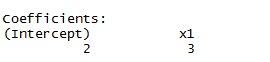beta: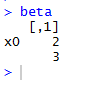Plot the best fit line:

abline(beta) or abline(fit)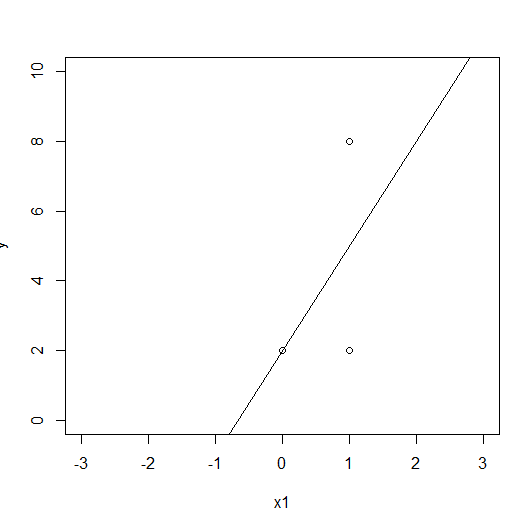We now have our best fit line drwan on our scatterplot btu now we want to find the coefficient of determination, denoted R2.

In order to calculate the R squared we need to calculate the “baseline prediction”, the “residual sum of squares (RSS)” and the “Total Sum of Squares (SST)”.

Baseline prediction is just is the average value of our dependent variable.(2+2+8)/3 = 4

The mean can also be computed in R as follows :

baseline <- mean(y)  or beasline <- sum(y)/nrow(y)

Residual sum of squares (RSS) or (SSR/SSE)  is the sum of the squares of residuals (deviations predicted from actual empirical values of data). It is a measure of the difference between the data and an estimation model. A small RSS indicates a good fit of the model to the data.Let’s implement the RSS in R:

#We first get all our values for f(xi)

Ypredict<-predict(fit,data.frame(x1))

#Then we compute the squared difference between y and f(xi) (Ypredict)

RSS<- sum((y – Ypredict)^2) #which gives ((2 – 2)^2 + (2 – 5)^2 + (8 – 5)^2) = 18

Total Sum of Squares (SST) or (TSS) is a statistical method which evaluates the sum of the squared difference between the actual X and the mean of X, from the overall mean.SST<-sum((y-baseline)^2) #baseline is the average of  y

We can now calculate the R squared:RSquare<-1 – (RSS/ SST) #which gives 1 – (18/24)=0.25

Part2: Quick way (without linear algebra)

data <- data.frame(x1=c(0, 1, 1), y = c(2, 2, 8))

plot(data, xlab=’x1′, ylab=’y’,xlim=c(-3,3), ylim=c(0,10))

fit <- lm(y~+x1)

abline(fit)

str(summary(fit)) # Will return many information included the R squared

/